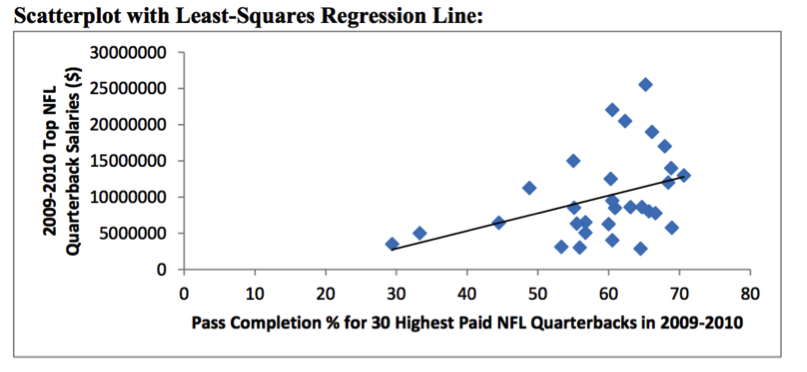# Descriptive Statistics

## Objective

Develop and answer statistical questions through data analysis of existing data using appropriate statistical measures and displays. (Part 3/3)

## Common Core Standards

### Core Standards

?

• HSS-ID.B.6 — Represent data on two quantitative variables on a scatter plot, and describe how the variables are related.

• HSS-ID.C.7 — Interpret the slope (rate of change) and the intercept (constant term) of a linear model in the context of the data.

• HSS-ID.C.8 — Compute (using technology) and interpret the correlation coefficient of a linear fit.

• HSS-ID.C.9 — Distinguish between correlation and causation.

?

• 8.SP.A.1

• 8.SP.A.2

• 8.SP.A.3

• 8.SP.A.4

## Criteria for Success

?

1. Develop a succinct yet informative written conclusion that clearly answers your statistical questions.
2. Identify calculations and other statistical evidence that support your solution.
3. Use statistical definitions to support your solution.
4. Use graphical analysis to support your solution.

## Tips for Teachers

?

• This is the third of three lessons that is project based. There is some guidance in the anchor problems that help to guide the project, but the data sets and questions can be swapped out.
• Additional data sets and options are included in the problem set guidance (same as in lesson 20).
• The target task for this lesson is a reflection of the work done in class and is not meant to be an assessment of how well students have completed this part of the project.

## Anchor Problems

?

Use the scatterplot, equation of the line of best fit, and correlation coefficient shown below to draw conclusions about your statistical question.${y=-4,443,755.85+244,351.32x}$

${r = 0.3940}$

#### References

STatistics Education Web (STEW) Grades 9-12, NFL Quarterback Salaries

## Problem Set

?

The following resources include problems and activities aligned to the objective of the lesson that can be used to create your own problem set.